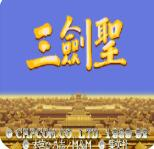# 三国志2三圣剑ROM中文加强版

三国志2三剑圣安卓最新版是游戏三国志2游戏加强版本之一，游戏中自带模拟器，经街机游戏下载中心测试过完全能运行。

## 可选角色

1.关羽：步行时没有拿兵器，以徒手战斗，绝技为猛虎扑。骑马时则执青龙偃月刀。

2.张飞：步行时没有拿兵器，以徒手战斗，有数种类似职业摔角的动作，绝技为暴裂拳。骑马时执丈八蛇矛。

3.赵云：步行时执剑，动作最敏捷，为唯一没有地面摔技的角色，但能空摔，绝技为升龙斩。骑马时执长枪。

4.黄忠：步行和骑马时皆执弓箭，为唯一能远距离攻击的角色，但不适合近战，绝技为冲天弓。

5.魏延：步行和骑马时皆执长刀，绝技为旋风腿。

## 出招表

关羽

A+B(去血保命招)﹔抓住敌人B，A(坐)﹔靠近敌人AAA(向前摔)﹔靠近敌人AATA(向后摔)↓1+A(大扑)↓+B(突进);马

上出招tA，→(向前摔)1A，一(向后摔)—→+A(蓝光)—→，B(红光)↓+B(下马)→+B(突进)→+B.B(旋风突进）连按A

(连打)

张飞

A+B(去血保命招)﹔抓住敌人B，A(坐);抓住敌人一或→B，A(反坐);抓住敌人↓+A(喝血);靠近敌人AA(!或)A(向前

摔)﹔靠近敌人AA(或t)HA(向后摔);靠近敌人↓→个不一+A(旋风大坐)↓f+A(冲拳)↓+B(突进)﹔马上出招tA，→(向

前摔)A，一(向后摔)—→+A(蓝光)—→，B(红光)↓+B(下马)→+B(突进)→+B.B(旋风突进）连按A(连打)

魏延

A+B(去血保命招)﹔抓住敌人B，A(坐)﹔靠近敌人AAIA(向前摔)﹔靠近敌人AATA(向后摔)↓+A(脚刀)↓+B(突进);马

上出招tA，→(向前摔)1A，一(向后摔)—→+A(蓝光)—→，B(红光)↓+B(下马)→+B(突进)→+B.B(旋风突进）连按A

(连打）

赵云

A+B(去血保命招)﹔抓住敌人B，A(侧踢）﹔靠近敌人AAtA(向前摔)﹔靠近敌人AAA(向后摔);↓t+A(通天剑);↓↓→产1+

A(滑步通天);↓+B(突进)(和敌人同时在空中);↓+A(空擒);马上出招tA，→(向前摔)tA，一(向后摔)—→+A(蓝光)一

→，B(红光)↓+B(下马）→+B(突进)→+B.B(旋风突进）连按A(连打)

黄忠

A+B(去血保命招)﹔连按A(三发箭)﹔抓住敌人B，A;(翻筋斗)↓+A;(旋转弓)↓+B;(突进)马上出招;一→+A(蓝光);

→，B(红光;）↓+B;(下马);→+B(突进);→+B.B(旋风突进)﹔连按A(三发箭)